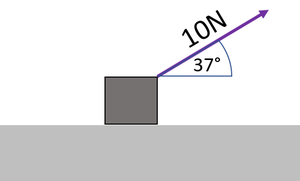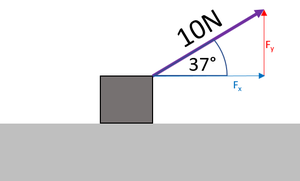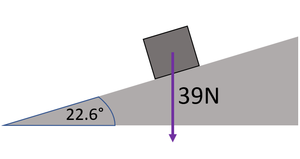# Resolving Forces

## Key Stage 4

### Meaning

To resolve a force is to separate a single force into two parts which act at right angles to one another.

### About Resolving Forces

Resolving forces is used to find how much of a force acts in a certain direction. For example:
It may be useful to know what 'component' of an object's weight acts down the slope to tell if is likely to accelerate down the slope.
A rope pulling at an angle above the horizontal has a vertical 'component' acting against weight and a horizontal 'component' acting to accelerate the object horizontally.

### ExamplesThe diagram gives the magnitude of the tension as 10N and the angle from the horizontal as 37°. Using the horizontal as the x-axis a right angle triangle is drawn with the tension as the hypotenuse to show the two perpendicular components. The component needed is the adjacent to the angle in the right angle triangle so cosine should be used. $$F_x = 10\cos(37)$$ $$F_x = 7.9863551N$$ $$F_x \approx 8.0N$$The diagram gives the magnitude of the weight as 39N and the angle of the slope as 22.6°. Using the slope as the x-axis a right angle triangle is drawn with the weight as the hypotenuse to show the two perpendicular components. The angle at the bottom of this triangle is the same as the angle of the slope. The component needed is the opposite to the angle in the right angle triangle so sine should be used. $$F_x = 39\sin(22.6)$$ $$F_x = 14.98751758N$$ $$F_x \approx 15N$$